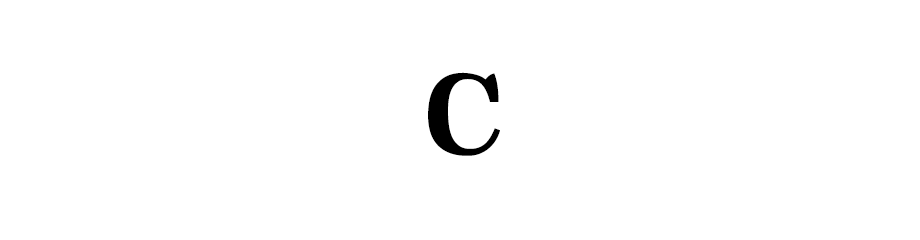# C - Tokens

C ProgrammingIn this tutorial we will learn about C tokens.

## Character Set

Its a set of characters that can be used to create values, variables, expressions etc.

Following are the characters that we can use in C programming language.

 LETTERS Uppercase A-ZLowercase a-z DIGITS All decimal digits 0-9 SPECIAL CHARACTERS `` ~ ! @ # \$ % ^ & * ( ) _ - + = { } | [ ] \ : " ; ' < > ? , . /` WHITE SPACE Blank Space, Horizontal tab, Carriage Return, New Line, Form feed

## What is Token?

The smallest individual unit in a program is known as token.

Following are the tokens used in C programming language.

• Keywords
• Identifiers
• Constants
• Strings
• Special Symbols
• Operators

## Keyword

Keywords are the words that conveys a special meaning to the language compiler and are reserved for special purpose and hence must not be used as normal identifier name.

### ANSI C KEYWORDS

There are 32 keywords.

 auto break case char const continue default do double else enum extern float for goto if int long register return short signed sizeof static struct switch typedef union unsigned void volatile while

## Identifiers

Identifiers are the fundamental building blocks of a program. They are used as the general terminology for the names givens to different parts of the program like the name of the variables, functions and arrays.

Identifiers name are user-defined and follow the following rules.

• First character of the identifier must be a letter or underscore
• Identifier names must only use letters (A-Z and a-z), digits (0-9) and underscore _
• Can't use white spaces
• Can't use keywords

Example: name, _gameStatus, is_game_over, stage99 etc.

## Constant

A value that will never change throughout the execution of the program is called a constant.

Example: 9.8 the value of g (acceleration due to gravity)

Following are the types of constants.

• Numeric Constants
• Integer Constants
• Real Constants
• Character Constants
• Single Character Constants
• Multi characters i.e., String Constants

### Integer Constants

Integers are numbers without decimal parts and there are three types of integers namely decimal, octal and hexadecimal integers.

Decimal integers are in base 10 number system and uses 10 digits from 0 to 9.

Example: -9, 0, 10 etc.

Octal integers are in base 8 number system and uses 8 digits from 0 to 7 with a leading 0.

Example: 07, 017 etc.

Hexadecimal integers are in base 16 number system and uses 10 digits from 0 to 9 and 6 letters from A to F with leading 0x and 0X. Lowercase letters from a to f can also be used.

Example: 0x11, 0xAA, 0xbb, 0x12F etc.

Click here for tutorial series on conversion of numbers from decimal to binary, octal, hexadecimal number system.

### Real Constants

These are the constants that have decimal parts and are also called as Floating Point Numbers.

Example: -10.234, 0, 3.14 etc.

We can also express real numbers in exponential form.

`mantissa e exponent`

Example: 1234 can be expressed in exponential form as `1.234e3`.

Similarly, -1200 can be expresses in exponential form as `-0.12e4`.

### Single Character Constant

A single character constant is a character enclosed in single quote.

Example: 'a', 'A', '1', '#' etc.

### ASCII value

Character constants have integer value known as the ASCII value.

If we want to print the ASCII value of a character we can use the `printf()` function.

``````printf("ASCII value of character 'A' is %d", 'A');
``````

The above code will give the following output:

ASCII value of character 'A' is 65

Similarly, we can use the ASCII value to print the character.

``````printf("Character for the ASCII value 65 is %c", 65);
``````

The above code will give us the following output.

Character for the ASCII value 65 is A

### Multi characters or String Constants

It is a sequence of characters enclosed in double quotes.

Example: "Hello World!"

A single character string constant like "A" does not have an ASCII value like a single character constant 'A'.

## String

A string is a sequence of characters enclosed in double quotes.

Example: "Hey" is a string consisting of three characters 'H', 'e' and 'y'.

## Special Symbols

Like `;` and `\$` etc.

## Operators

Operators are the symbols that are meant to perform some operation.

Example: `+` is an addition operator and we use it to add two numbers i.e., perform addition operation.

## Escape Sequence

These are special backslash character constants.

Character ConstantDescription
'\0'null
'\n'New line
'\''Single quote
'\"'Double quotes
``````printf("Hello World\n");